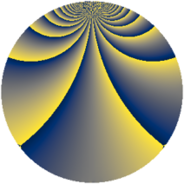# Properties

 Label 441.2.bdLevel $441$ Weight $2$ Character orbit 441.bd Rep. character $\chi_{441}(47,\cdot)$ Character field $\Q(\zeta_{42})$ Dimension $648$ Newform subspaces $1$ Sturm bound $112$ Trace bound $0$

# Related objects

## Defining parameters

 Level: $$N$$ $$=$$ $$441 = 3^{2} \cdot 7^{2}$$ Weight: $$k$$ $$=$$ $$2$$ Character orbit: $$[\chi]$$ $$=$$ 441.bd (of order $$42$$ and degree $$12$$) Character conductor: $$\operatorname{cond}(\chi)$$ $$=$$ $$441$$ Character field: $$\Q(\zeta_{42})$$ Newform subspaces: $$1$$ Sturm bound: $$112$$ Trace bound: $$0$$

## Dimensions

The following table gives the dimensions of various subspaces of $$M_{2}(441, [\chi])$$.

Total New Old
Modular forms 696 696 0
Cusp forms 648 648 0
Eisenstein series 48 48 0

## Trace form

 $$648 q - 18 q^{2} - 11 q^{3} - 60 q^{4} - 15 q^{5} - 22 q^{6} - 5 q^{7} + 13 q^{9} + O(q^{10})$$ $$648 q - 18 q^{2} - 11 q^{3} - 60 q^{4} - 15 q^{5} - 22 q^{6} - 5 q^{7} + 13 q^{9} - 22 q^{10} - 21 q^{11} + 24 q^{12} - 10 q^{13} - 24 q^{14} - 10 q^{15} + 42 q^{16} + 9 q^{17} + 12 q^{18} - 36 q^{19} - 15 q^{20} - 38 q^{21} - 9 q^{22} - 84 q^{23} - 38 q^{24} - 97 q^{25} - 6 q^{26} - 20 q^{27} - 26 q^{28} - 123 q^{29} - q^{30} - 6 q^{31} - 30 q^{32} - 8 q^{33} - q^{34} + 70 q^{36} - 13 q^{37} - 75 q^{38} - 24 q^{39} - 7 q^{40} - 15 q^{41} - 133 q^{42} - 9 q^{43} - 51 q^{44} - 41 q^{45} - 108 q^{46} - 36 q^{47} + 15 q^{48} + 5 q^{49} - 45 q^{50} + 10 q^{51} - 35 q^{52} + 12 q^{53} - 50 q^{54} + 14 q^{55} + 33 q^{56} - 7 q^{57} + 33 q^{58} - 3 q^{59} + 180 q^{60} + 55 q^{61} - 66 q^{62} - 20 q^{63} + 64 q^{64} + 3 q^{65} + 285 q^{66} + 13 q^{67} + 6 q^{68} + 82 q^{69} + 11 q^{70} + 63 q^{71} - 21 q^{72} - 22 q^{73} - 21 q^{74} - 107 q^{75} + 21 q^{76} - 69 q^{77} + 63 q^{78} + q^{79} + 45 q^{80} - 211 q^{81} - 28 q^{82} + 9 q^{83} + 239 q^{84} - 10 q^{85} - 21 q^{86} - 109 q^{87} - 29 q^{88} - 132 q^{89} - 275 q^{90} - 13 q^{91} + 195 q^{92} + 19 q^{93} - 4 q^{94} - 129 q^{95} - 5 q^{96} - 3 q^{97} - 21 q^{98} - 148 q^{99} + O(q^{100})$$

## Decomposition of $$S_{2}^{\mathrm{new}}(441, [\chi])$$ into newform subspaces

Label Dim. $$A$$ Field CM Traces $q$-expansion
$$a_2$$ $$a_3$$ $$a_5$$ $$a_7$$
441.2.bd.a $$648$$ $$3.521$$ None $$-18$$ $$-11$$ $$-15$$ $$-5$$